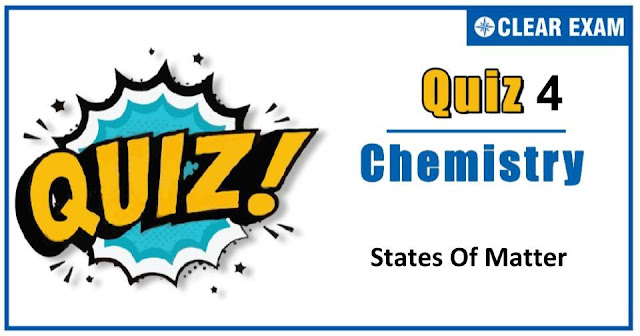## States of Matter Quiz-4

The one subject in NEET which is candidates who can easily attain good marks is Chemistry. That's the reason, often, one doesn’t pay notice and choose to compromise it. But if one wants to rank above others, the tip is to be thorough with NEET chemistry concepts. The understanding of reactions and definite basic understanding is what requires major attention in Chemistry but once done it only gets simpler from there. The main focus on the to-do list should be on getting a hang of the NCERT syllabus of NEET chemistry..

Q1. The molecular velocities of two gases at the same temperature are u1 and u2 and their masses are m1 and m2 respectively. Which of the following expressions are correct?
•  m1/u12 =m2/u22
•  m1 u1=m2 u2
•  m1/u1 =m2/u2
•  m1 u12=m2 u22
Solution
(d) u1/u2 =√(m2/m1 ×T1/T2 ) ∵ T1=T2 So,(u12)/(u22 )=m2/m1 or u12 m1=u22 m2

Q2.According to kinetic theory of gases for a diatomic molecule
•  The pressure exerted by the gas is proportional to the mean square speed of the molecules
•  The pressure exerted by the gas is proportional to the root mean square speed of the molecules
•  The root mean square speed is inversely proportional to the temperature
•  The mean translational KE of the molecule is directly proportional to the absolute temperature
Solution
(d) The mean translational KE of the molecule is directly proportional to the absolute temperature

Q3. The kinetic theory of gases predicts that total kinetic energy of a gaseous assembly depends on
•  Pressure of the gas
•  Temperature of the gas
•  Volume of the gas
•  Pressure, volume and temperature of the gas
Solution
(b) The average kinetic energy of a gaseous assembly depends on temperature of the gas KE ∝T

Q4. The flame colours of metal ions are due to
•  Schottky defect
•  Frenkel defect
•  Metal excess defect
•  Metal deficiency defect
Solution
(c) In metal excess defect when holes created by missing of anions are occupied by electrons, there sites are called F-centres and are responsible for colour in the crystal

Q5.When two atoms of hydrogen combine to form a molecule of hydrogen gas, the energy of the molecule is:
•  Equal to that of sum of energy of separate atoms
•  Higher than that of sum of energy of separate atoms
•  Lower than that of sum of energy of separate atoms
•  None of the above
Solution
(c) Bond formation is exothermic.

Q6. The solid NaCl is a bad conductor of electricity since
•  In solid NaCl, there is no velocity of ions
•  In solid NaCl, there are no ions
•  In solid NaCl, there are no electrons
•  Solid NaCl is covalent
Solution
(a) Solid NaCl is a bad conductor of electricity because ions are not free to move

Q7.Which one of the following volume (V)-temperature (T) plots represents the behaiour of one mole of an ideal gas at one atmospheric pressure?
Solution
(c) V-T plot representing the behavior of 1 mole of an ideal gas at 1 atm pressure. Volume of 1 mole of an ideal gas at 273 K and 1 atm pressure is 22.4 L. Volume of 1 mole of an ideal gas at 373 K and 1 atm pressure will be V=RT/p=(0.0821×373)/1=30.58 L

Q8.Which gas contains larger number of molecules?
•  4 g of H2 O
•  2 g of marsh gas
•  4 g of PCl5
•  2 g of phoszene
Solution
(a) More is the number of mole, more will be number of molecules.

Q9.If a gas is expanded at constant temperature:
•  Number of molecules of the gas decreases
•  The kinetic energy of the molecules decreases
•  The kinetic energy of the molecules remains the same
•  The kinetic energy of the molecules increases
Solution
(c) Kinetic energy depends on temperature only.

Q10. Which statement about evaporation is incorrect?
•  Evaporation takes place at all temperature
•  Evaporation occurs only at the surface
•  Evaporation produces cooling
•  Average KE of residual liquid molecules increase as evaporation occurs
Solution
(d) Evaporation takes place at constant temperature and thus, kinetic energy does not change.#### Written by: AUTHORNAME

AUTHORDESCRIPTION## Want to know more

Please fill in the details below:

## Latest NEET Articles\$type=three\$c=3\$author=hide\$comment=hide\$rm=hide\$date=hide\$snippet=hide

Name

ltr
item
BEST NEET COACHING CENTER | BEST IIT JEE COACHING INSTITUTE | BEST NEET & IIT JEE COACHING: states-of-matter-quiz-4
states-of-matter-quiz-4
https://1.bp.blogspot.com/-4QX9jiHZBYw/X5gpzEWymnI/AAAAAAAAF9s/abGWwd4CDNgQiqRYHfHIJ8RXf9WwTRYgQCLcBGAsYHQ/w640-h336/Quiz%2BImage%2B20%2B%25283%2529.jpg
https://1.bp.blogspot.com/-4QX9jiHZBYw/X5gpzEWymnI/AAAAAAAAF9s/abGWwd4CDNgQiqRYHfHIJ8RXf9WwTRYgQCLcBGAsYHQ/s72-w640-c-h336/Quiz%2BImage%2B20%2B%25283%2529.jpg
BEST NEET COACHING CENTER | BEST IIT JEE COACHING INSTITUTE | BEST NEET & IIT JEE COACHING
https://www.cleariitmedical.com/2020/10/states-of-matter-quiz-4.html
https://www.cleariitmedical.com/
https://www.cleariitmedical.com/
https://www.cleariitmedical.com/2020/10/states-of-matter-quiz-4.html
true
7783647550433378923
UTF-8

STAY CONNECTED